# Lightwave System Design Guidelines

The design of fiber-optic communication systems requires a clear understanding of the limitations imposed by the loss, dispersion, and nonlinearity of the fiber. Since fiber properties are wavelength dependent, the choice of operating wavelength is a major design issue. In this tutorial, we discuss how the bit rate and the transmission distance of a single-channel system are limited by fiber loss and dispersion. In the next tutorial we will discuss multichannel systems. We also consider the power and rise-time budgets and illustrate them through specific examples. The power budget is also called the link budget, and the rise-time budget is sometimes referred to as the bandwidth budget.

#### 1. Loss-Limited Lightwave Systems

Except for some short-haul fiber links, fiber losses play an important role in the system design. Consider an optical transmitter that is capable of launching an average power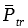. If the signal is detected by a receiver that requires a minimum average powerat the bit rate B, the maximum transmission distance is limited bywhere αf is the net loss (in dB/km) of the fiber cable, including splice and connector losses. The bit-rate dependence of L arises from the linear dependence ofon the bit rate B. Noting that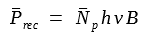, where hν is the photon energy andis the average number of photons/bit required by the receiver, the distance L decreases logarithmically as B increases at a given operating wavelength. Now let's take a look at the figure below.The solid lines in the figure above show the dependence of L on B for three common operating wavelengths of 0.85, 1.3, and 1.55 μm by using αf = 2.5, 0.4, and 0.25 dB/km, respectively. The transmitted power is taken to be= 1 mW at the three wavelengths, whereas= 300 at λ = 0.85 μm and= 500 at 1.3 and 1.55 μm. The smallest value of L occurs for first-generation systems operating at 0.85 μm because of relatively large fiber losses near that wavelength. The repeater spacing of such systems is limited to 10-25 km, depending on the bit rate and the exact value of the loss parameter. In contrast, a repeater spacing of more than 100 km is possible for lightwave system operating near 1.55 μm.

It is interesting to compare the loss limit of 0.85-μm lightwave systems with that of electrical communication systems based on coaxial cables. The dotted line in the figure above shows the bit-rate dependence of L for coaxial cables by assuming that the loss increases as. The transmission distance is larger for coaxial cables at small bit rates (B < 5 Mb/s), but fiber-optic systems take over at bit rates in excess of 5 Mb/s. Since a longer transmission distance translates into a smaller number of repeaters in a long-haul point-to-point link, fiber-optic communication systems offer an economic advantage when the operating bit rate exceeds 10 Mb/s.

The system requirements typically specified in advance are the bit rate B and the transmission distancer L. The performance criterion is specified through the BER, a typical requirement being BER < 10-9.  The first decision of the system designer concerns the choice of the operating wavelength. As a practical matter, the cost of components is lowest near 0.85 μm and increases as wavelength shifts toward 1.3 and 1.55 μm.

The above figure can be quite helpful in determining the appropriate operating wavelength. Generally speaking, a fiber-optic link can operate near 0.85 μm if B < 200 Mb/s and L < 20 km. This is the case for many LAN applications. On the other hand, the operating wavelength is by necessity in the 1.55-μm region for long-haul lightwave systems operating at bit rates in excess of 2 Gb/s. The curves shown in the figure provide only a guide to the system design. Many other issues need to be addressed while designing a realistic fiber-optic communication system. Among them are the choice of operating wavelength, selection of appropriate transmitters, receivers, and fibers, and the issues of cost, performance, and system reliability.

#### 2. Dispersion-Limited Lightwave Systems

In the previous tutorials, we discussed how fiber dispersion limits the bit rate-distance product BL because of pulse broadening. When the dispersion-limited transmission distance is shorten than the loss-limited distance, the system is said to be dispersion-limited. The dashed lines in the figure above show the dispersion-limited transmission distance as a function of the bit rate. Since the physical mechanisms leading to dispersion limitation can be different for different operating wavelengths, let us examine each case separately.

Consider first the case of 0.85-μm lightwave systems, which often use multimode fibers to minimize the system cost. As discussed in the previous tutorial, the most limiting factor for multimode fibers is intermodal dispersion. In the case of step-index multimode fibers, this equation provides an upper bound on the BL product: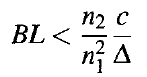It is plotted in the figure above using n1 = 1.46 and Δ = 0.01. Even at a low bit rate of 1 Mb/s, such multimode systems are dispersion-limited, and their transmission distance is limited to below 10 km. For this reason, multimode step-index fibers are rarely used in the design of fiber-optic communication systems. Considerable improvement can be realized by using graded-index fibers for which intermodal dispersion limits the BL product to values given by the following equation:The condition BL = 2c(n1Δ2) is plotted in the figure above and shows that 0.85-μm lightwave systems are loss-limited, rather than dispersion-limited, for bit rates up to 100 Mb/s when graded-index fibers are used. The first generation of terrestrial system took advantage of such an improvement and used graded-index fibers. The first commercial system became available in 1980 and operated at a bit rate of 45 Mb/s with a repeater spacing of less than 10 km.

The second generation of lightwave systems used primarily single-mode fibers near the minimum-dispersion wavelength occurring at about 1.31 μm. The most limiting factor for such systems is dispersion-induced pulse broadening dominated by a relatively large source spectral width. As discussed in previous tutorial, the BL product is then limited by the following equation: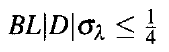The value of |D| depends on how close the operating wavelength is to the zero-dispersion wavelength of the fiber and is typically ~ 1 ps/(km-nm). The figure above shows the dispersion limit for 1.3-μm lightwave systems by choosing |D|σλ = 2 ps/km so that BL <= 125 (Gb/s)-km. As seen there, such systems are generally loss-limited for bit rates up to 1 Gb/s but become dispersion-limited at higher bit rates.

Third- and fourth-generation lightwave systems operate near 1.55 μm to take advantage of the smallest fiber losses occurring in this wavelength region. However, dispersion becomes a major problem for such systems because D ≈ 16 ps/(km-nm) near 1.55 μm for standard silica fibers. Semiconductor lasers operating in a single longitudinal mode provide a solution to this problem. The dispersion limit is then given by this equation: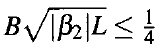The figure above shows this limit by choosing B2L = 4000 (Gb/s)2-km. As seen there, such 1.55-μm systems become dispersion-limited only for B > 5 Gb/s. In practice, the frequency chirp imposed on the optical pulse during direct modulation provides a much m ore severe limitation. Qualitatively speaking, the frequency chirp manifests through a broadening of the pulse spectrum. If we use the following equation:with D = 16 ps/(km-nm) and σλ = 0.1 nm, the BL product is limited to 150 (Gb/s)-km. As a result, the frequency chirp limits the transmission distance to 75 km at B = 2 Gb/s, even though loss-limited distance exceeds 150 km. The frequency-chirp problem is often solved by using an external modulator for systems operating at bit rates > 5 Gb/s.

A solution to the dispersion problem is offered by dispersion-shifted fibers for which dispersion and loss both are minimum near 1.55 μm. The figure above shows the improvement by usingwith |β2| = 2 ps2/km. Such systems can be operated at 20 Gb/s with a repeater spacing of about 80 km. Further improvement is possible by operating the lightwave system very close to the zero-dispersion wavelength, a task that is not always feasible because of variations in the dispersive properties of the fiber along the transmission link. In practice, the frequency chirp makes it difficult to achieve even the limit indicated in the figure. By 1989, two laboratory experiments had demonstrated transmission over 81 km at 11 Gb/s and over 100 km at 10 Gb/s by using low-chirp semiconductor lasers together with dispersion-shifted fibers. The triangles in the figure shows that such systems operate quite close to the fundamental limits set by fiber dispersion. Transmission over longer distances requires the use of dispersion management, a technique we will discuss in future tutorials.

#### 3. Power Budget

The purpose of the power budget is to ensure that enough power will reach the receiver to maintain reliable performance during the entire system lifetime. The minimum average power required by the receiver is the receiver sensitivity. The average launch poweris generally known for any transmitter. The power budget takes an especially simple form in decibel units with optical power expressed in dBm units. More specifically,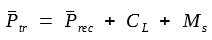where CL is the total channel loss and Ms is the system margin. The purpose of system margin is to allocate a certain amount of power to additional sources of power penalty that may develop during system lifetime because of component degradation or other unforeseen events. A system margin of 3-4 dB is typically allocated during the design process.

The channel loss CL should take into account all possible sources of power loss, including connector and splice losses. If αf  is the fiber loss in decibels per kilometer, CL can be written as

CL = αfL + αcon + αsplice

where αcon and αsplice account for the connector and splice losses throughout the fiber link.  Sometimes splice loss is included within the specified loss of the fiber cable. The connector loss αcon includes connectors at the transmitter and receiver ends but must include other connectors if used within the fiber link.

The two equations above can be used to estimate the maximum transmission distance for a given choice of components. As an illustration, consider the design of a fiber link operating at 100 Mb/s and requiring a maximum transmission distance of 8 km. As seen from the figure above, such a system can be designed to operate near 0.85 μm provided that a graded-index multimode fiber is used for the optical cable. The operation near 0.85 μm is desirable from the economic standpoint. Once the operating wavelength is selected, a decision must be made about the appropriate transmitters and receivers. The GaAs transmitter can use a semiconductor laser or an LED as an optical source. Similarly, the receiver can be designed to use either a p-i-n or an avalanche photodiode. Keeping the low cost in mind, let us choose a p-i-n receiver and assume that it requires 2500 photons/bit on average to operate reliably with a BER below 10-9. Using the relationwith= 2500 and B = 100 Mb/s, the receiver sensitivity is given by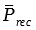= -42 dBm. The average launch power for LED and laser-based transmitters is typically 50 μW and 1 mW, respectively.

The table below shows the power budget for the two transmitters by assuming that the splice loss is included within the cable loss. The transmission distance L is limited to 6 km in the case of LED-based transmitters. If the system specification is 8 km, a more expensive laser-based transmitter must be used. The alternative is to use an avalanche photodiode (APD) receiver. If the receiver sensitivity improves by more than 7 dB when an APD is used in place of a p-i-n photodiode, the transmission distance can be increased to 8 km even for an LED-based transmitter. Economic considerations would then dictate the choice between the laser-based transmitters and APD receivers.#### 4. Rise-Time Budget

The purpose of the rise-time budget is to ensure that the system is able to operate properly at the intended bit rate. Even if the bandwidth of the individual system components exceeds the bit rate, it is still possible that the total system may not be able to operate at that bit rate. The concept of rise time is used to allocate the bandwidth among various components. The rise time Tr of a linear system is defined as the time during which the response increases from 10 to 90% of its final output value when the input is changed abruptly. The following figure illustrates the concept graphically.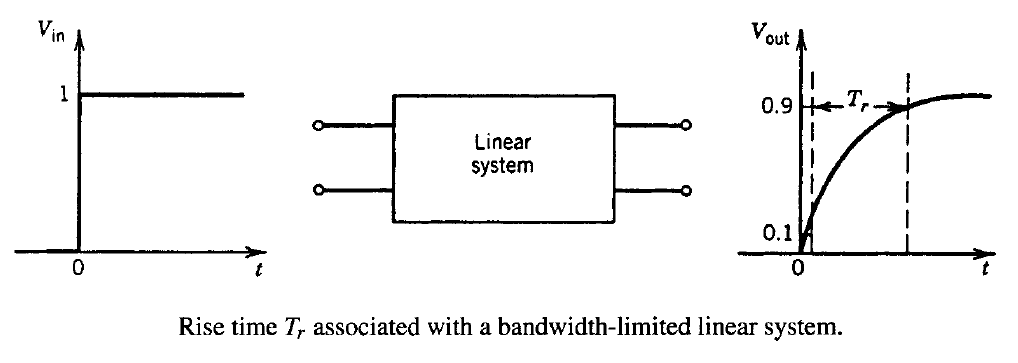An inverse relationship exists between the bandwidth Δf and the rise time Tr associated with a linear system. This relationship can be understood by considering a simple RC circuit as an example of the linear system. When the input voltage across an RC circuit changes instantaneously from 0 to V0, the output voltage changes as

Vout(t) = V0[1 - exp(-t/RC)]

where R is the resistance and C is the capacitance of the RC circuit. The rise time is found to be given by

Tr = (ln9)RC ≈ 2.2RC

The transfer function H(f) of the RC circuit is obtained by taking the Fourier transform of the Vout(t) equation and is of the form

H(f) = (1 + i2πfRC)-1

The bandwidth Δf of the RC circuit corresponds to the frequency at which |H(f)|2 = 1/2 and is given by the well known expression Δf = (2πRC)-1. By using Tr equation above, Δf and Tr are related asThe inverse relationship between the rise time and the bandwidth is expected to hold for any linear system. However, the product TrΔf would generally be different than 0.35. One can use TrΔf = 0.35 in the design of optical communication systems as a conservative guideline. The relationship between the bandwidth Δf and the bit rate B depends on the digital format. In the case of return-to-zero (RZ) format, Δf = B and BTr = 0.35. By contrast, Δf ≈ B/2 for the nonreturn-to-zero (NRZ) format and BTr = 0.7. In both cases, the specified bit rate imposes an upper limit on the maximum rise time that can be tolerated. The communication system must be designed to ensure that Tr is below this maximum value, i.e.,The three components of fiber-optic communication systems have individual rise times. The total rise time of the whole system is related to the individual component rise times approximately as

Tr2 = Ttr2 + Tfiber2 + Trec2

where Ttr, Tfiber, and Trec are the rise times associated with the transmitter, fiber, and receiver, respectively. The rise times of the transmitter and receiver are generally known to the system designer. The transmitter rise time Ttr is determined primarily by the electronic components of the driving circuit and the electrical parasitics associated with the optical source. Typically, Ttr is a few nanoseconds for LED-based transmitters but can be shorter than 0.1 ns for laser-based transmitters. The receiver rise time Trec is determined primarily by the 3-dB electrical bandwidth of the receiver front end.  This same equation can be used to estimate Trec if the front-end bandwidth is specified.The fiber rise time Tfiber should in general include the contributions of both the intermodal dispersion and the group-velocity dispersion (GVD) through the relation

Tfiber2 = Tmodal2 + TGVD2

For single-mode fibers, Tmodal = 0 and Tfiber =  TGVD. In principle, one can use the concept of fiber bandwidth and relate Tfiber to the 3-dB fiber bandwidth f3 dB. In practice, it is not easy to calculate f3 dB, especially in the case of modal dispersion. The reason is that a fiber link consists of many concatenated fiber sections (typical length 5 km), which may have different dispersion characteristics. Furthermore, mode mixing occurring at splices and connectors tends to average out the propagation delay associated with different modes of a multimode fiber. A statistical approach is often necessary to estimate the fiber bandwidth and the corresponding rise time.

In a phenomenological approach, Tmodal can be approximated by the time delay ΔT in the absence of mode mixing, i.e.,

Tmodal ≈ (n1Δ/c)L

where n1  n2 was used. For graded-index fibers, resulting in Tmodal ≈ (n1Δ2/8c)L. In both cases, the effect of mode mixing is included by changing the linear dependence on L by a sublinear dependence Lq, where q has a value in the range 0.5-1, depending on the extent of mode mixing. A reasonable estimate based on the experimental data is q = 0.7. The contribution TGVD can also be approximated by ΔT, so that

TGVD ≈ |D|LΔλ

where Δλ is the spectral width of the optical source (taken as a full width at half maximum). The dispersion parameter D may change along the fiber link if diferent sections have different dispersion characteristics; an average value should be used in that case.

As an illustration of the rise-time budget, consider a 1.3-μm lightwave system designed to operate at 1 Gb/s over a single-mode fiber with a repeater spacing of 50 km. The rise times for the transmitter and the receiver have been specified as Ttr = 0.25 ns and Trec = 0.35 ns. The source spectral width is specified as Δλ = 3 nm, whereas the average value of D is 2 ps/(km-nm) at the operating wavelength. From the equation above, TGVD = 0.3 ns for a link length L = 50 km. Modal dispersion does not occur in single-mode fibers. Hence Tmodal = 0 and Tfiber = 0.3 ns. The system rise time is estimated by using Tr2 = Ttr2 + Tfiber2 + Trec2 and is found to be Tr = 0.524 ns. The use ofindicates that such a system cannot be operated at 1 Gb/s when the RZ format is employed for the optical bit stream. However, it would operate properly if digital format is changed to the NRZ format. If the use of RZ format is a prerequisite, the designer must choose different transmitters and receivers to meet the rise-time budget requirement. The use of the NRZ format was prevalent during the 1990s, but the RZ format has become the format of choice for long-haul systems operating at a bit rate of 40 Gb/s, especially when the information is coded in the phase of the optical carrier.